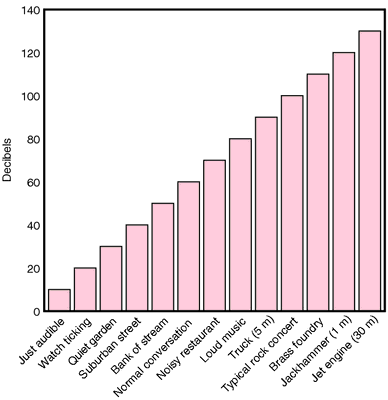# decibel

(redirected from Line level)
Also found in: Dictionary, Encyclopedia, Wikipedia.

## decibel

[des´ĭ-bel]
a unit of relative power intensity equal to one tenth of a bel, used for electric or acoustic power measurements; one decibel equals approximately the smallest difference in acoustic power the human ear can detect and an increase of 10 decibels approximately doubles the loudness of a sound. Abbreviated dB or db.Examples of decibel levels in everyday situations. From Frazier et al., 1996.

## dec·i·bel (dB, db),

(des'i-bel), Avoid the mispronunciation des'i-b'l.
One tenth of a bel; unit for expressing the relative intensity of sound on a logarithmic scale.
[L. decimus, tenth, + bel]

## decibel

/dec·i·bel/ (des´ĭ-bel) a unit used to express the ratio of two powers, usually electric or acoustic powers, equal to one-tenth of a bel; one decibel equals approximately the smallest difference in acoustic power the human ear can detect.

## decibel (dB)

[des′əbəl]
Etymology: L, decimus, one tenth, bel, Alexander G. Bell, Canadian inventor, 1847-1922
a unit of measure of the intensity of sound. A decibel is one tenth of 1 bel (B); an increase of 1 B is perceived as a 10-fold increase in loudness, based on a sound-pressure reference level of 0.0002 dyne/cm2, or 20 micropascals.

## dec·i·bel

(dB) (des'i-bĕl)
One tenth of a bel; unit for expressing the relative loudness of sound on a logarithmic scale.
[L. decimus, tenth, + bel]

## decibel

A logarithmic unit of comparison between a standard power level and an observed level. The decibel is not a unit of sound intensity but is widely used to compare a noise level with a very low standard reference level near the limit of audibility, and to compare electrical power levels. A tenth of a bel.

## Decibel

A unit of measure for expressing the loudness of a sound. Normal speech is typically spoken in the range of about 20-50 decibels.
Mentioned in: Audiometry, Hearing Loss

## decibel (dB)

1. Unit used for the measurement of the intensity of a sound. 2. Light intensities are often presented on a logarithmic (rather than linear) scale. This is done, in particular, to abbreviate large numbers. Moreover, it has become common, especially in perimetry, to use decibels rather than log units. A decibel scale is a logarithmic scale where 10 decibels are equal to 1 log unit; 20 decibels, to 2 log units, etc. In perimetry, decibels are used to indicate the attenuation of brightness of the stimulus. Thus, a 20 dB stimulus is equal to one-tenth the brightness of a 10 dB stimulus.

## dec·i·bel

(dB) (des'i-bĕl)
One tenth of a bel.
[L. decimus, tenth, + bel]

## decibel

a unit used to express the ratio of two powers, usually electric or acoustic powers, equal to one-tenth of a bel; one decibel equals approximately the smallest difference in acoustic power the human ear can detect. Abbreviated dB or db. See also bel.
Site: Follow: Share:
Open / Close